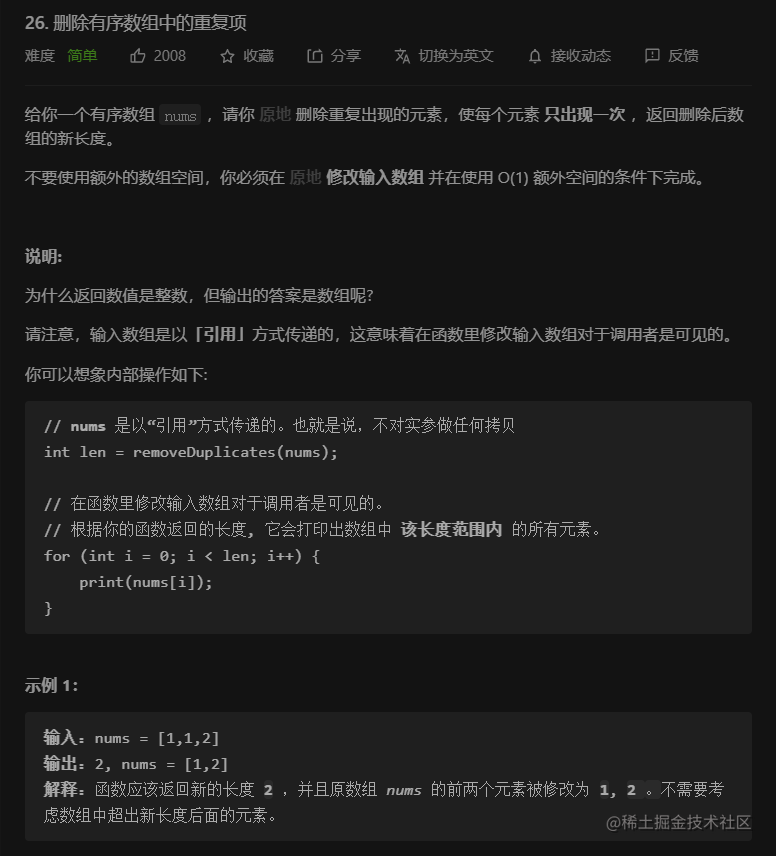# LeetCode 26. 删除有序数组中的重复项：题解｜刷题打卡

## 一、题目描述：## 三、AC 代码：

class Solution {
public:
int removeDuplicates(vector<int>& nums) {
int l = 0, r = 0, n = nums.size();
while (r < n) {
int val = nums[r];
while (r < n && nums[r] == val) {
++r;
}
nums[l++] = val;
}
return l;
}
};